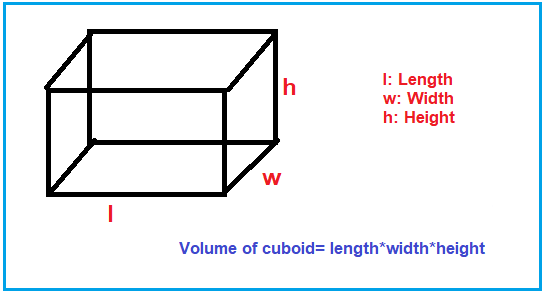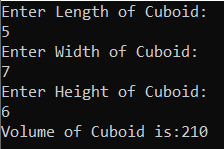# Volume of Cuboid in C#

## Volume of Cuboid in C# with Examples

In this article, I am going to discuss how to calculate the Volume of Cuboid in C# with Examples. Please read our previous article, where we discussed How to Calculate the Surface Area of Cube in C#. Here, in this article, first, we will discuss what is Cuboid, then we will discuss how to calculate the Volume of Cuboid and finally, we will see how to implement the Volume of Cuboid program in C#.

##### What is a Cuboid?

A cuboid is a 3D (3-dimensional plane). A cuboid has 6 rectangle-shaped faces, 8 vertices, and 12 edges. Each face meets another face at 90 degrees each. it has length, width, and height. The volume of an object is defined as the amount of space that a solid occupies. Volume of cuboid= length*width*height. For better understanding, please have a look at the following diagram.##### Example: in cm

Input: length=7cm, width=5cm, height=2cm
Output: Volume of Cuboid =height*width *length
= (7×5×2)
= 70 cm
3

In this program, we are going to use the following Data types,

1. Float: Floating-point numbers are used for decimal and exponential values. It is a primitive data type. It has 6 decimal digits of precision. Example: variables float b = 6.3
2. Double: Double is also a data type that is used to represent the floating-point numbers. It has 15 decimal digits of precision. Example: double y = 2345.234
##### Logic to Calculate the Volume of Cuboid in C#

To calculate the Volume of Cuboid, we need to follow the below steps

1. First, we will define variables, length, width, height, volume, and assign the value of length, width, height in it.
2. After that, we will calculate the volume of the cuboid by formula i.e. volume=height*width *length
3. Then calculated value store in volume (variable).
4. At last, the result will show as the output.
##### Example: Volume of Cuboid Program in C#.

The following C# Program will calculate the Volume of Cuboid.

```using System;
class VolumeaOfCuboid
{
static void Main()
{
float length, width, height;
length = 5;
width = 3;
height = 4;
float volume = height * width * length;
Console.WriteLine("Volume of Cuboid is:" + volume);
}
}
```

Output: Volume of Cuboid is:60

##### Example: Taking input from the user

In the following C# program, we will take the Cuboid length, width, and height from the user and then calculate the Volume and print it on the console.

```using System;
class VolumeaOfCuboid
{
static void Main()
{
Console.WriteLine("Enter Length of Cuboid:");
Console.WriteLine("Enter Width of Cuboid:");
Console.WriteLine("Enter Height of Cuboid:");
double Volume = Height * Width * Length;
Console.WriteLine("Volume of Cuboid is:" + Volume);
}
}
```
###### Output:##### C# Code (Using function)

In the below example, we created one method to calculate the Volume of Cuboid.

```using System;
class VolumeaOfCuboid
{
static void Main()
{
Console.WriteLine("Enter Length of Cuboid:");
Console.WriteLine("Enter Width of Cuboid:");
Console.WriteLine("Enter Height of Cuboid:");
double Volume = CalculateVolume(Length, Width, Height);

Console.WriteLine("Volume of Cuboid is:" + Volume);